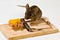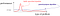# The No Free Lunch Theorem (or why you can’t have your cake and eat it)

In this series I’ll be taking a random walk through the algorithms and ideas that I learn from my Natural Computing class that I’m taking at Goldsmiths, University of London.

Please find part two, part three and part four of the series here!

Where possible, I’ll be combining the topical material with a deep learning / connectionist slant to complement the work I’ll be doing elsewhere this year.

The No Free Lunch Theorem (NFLT) is named after the phrase, there ain’t no such thing as a free lunch. As a pretext to this article, it is worth exploring the meaning of this phrase. There is/ain’t no such thing as free lunch has origins in the mid-nineteenth century onwards, whereby bar and saloon owners would attract drinkers in with free food on condition that they brought a drink, whereby the working class customer would likely be better off not drunk and with the money in their pocket.Fig 1. Unfortunately, there is no such thing as free lunch.

There are a few things we need to review before getting into the guts of the NFLT. If you are like me and generally baulk at mathematics, feel free to skip to the higher level section further down this article.

Firstly, we are dealing with comparison of optimisation problems, sometimes called ‘cost functions’ or ‘energy functions’, which are represented as a mapping between points in a search space and the points in the space of possible ‘fitness’ or ‘cost’ values. Note that NFLT also stands for search algorithms, but the specifics will not be covered in this article.

Next, there are a few conditionals of NFLT:

1. The search space the optimiser iterates through will be finite.
2. The space of possible cost values will also be finite.

These two conditions are automatically met for optimisation algorithms ran on any computer; even if the potential values are floating point variables there is still a discrete range of values due to how floating point values are represented on a computer. Because the size of the two spaces are are both finite, this means the size of the set of all possible problems are finite.

Probability theory was utilised so that David H. Wolpert and William G. Macready could generalise their results for both stochastic and deterministic algorithms.

Here, ‘dₘ’ is the set of size ‘m’ of the cost values ‘y’. The ‘y’ cost values are associated to the aforementioned mapping (using the cost function ‘f’) of the search space and the cost values. This above statement gives us the probability of getting a sequence of cost values from the algorithm ‘a’ iterated ‘m’ times using the cost function ‘f’. Using this, Wolpert and Macready went on to state their first theorem:

The above theorem (the proof found in No Free Lunch Theorems for Optimisation) shows a few things. For the pair of algorithms ‘a₁’ and ‘a₂’ in the above equation, we can firstly, and crucially, note that the average of performance is independent of the algorithm. This theorem is demonstrating what a given algorithm gains on one of class problems it is equally offset by its performance on the remaining problems.

In their paper, Wolpert and Macready give a second NFLT, which is analogous to the first but shows that objective functions that vary with time. For brevity and my own sanity I’ve omitted this from the article. This second theorem means if an algorithm outperforms another with certain kinds of cost function dynamics, then the reverse must be true on the set of all other cost function dynamics.

The NLFT are a set of mathematical proofs and general framework that explores the connection between general-purpose algorithms that are considered “black-box” and the problems they solve.

The No Free Lunch Theorems state that any one algorithm that searches for an optimal cost or fitness solution is not universally superior to any other algorithm.

This is due to the huge potential problem space for the application of the general-purpose algorithm; if an algorithm is particularly adept at solving one class of problem, and the fitness surface that comes with it, then then it has to perform worse on the remaining average of problems. Wolpert and Macready wrote in their paper, No Free Lunch Theorems for Optimisation:

“If an algorithm performs better than random search on some class of problems then in must perform worse than random search on the remaining problems.”

In short because of the NFLT proof, we can state: A superior black-box optimisation strategy is impossible.

There is another issue to consider, which is the generality versus the specificity of the algorithm. This means we must weigh the tradeoff between an efficient algorithm which is very specific in the space of problems it can address, or the general algorithm which a jack of all trades, but a master of none.Fig 2. Theorem one shows us that the use of domain knowledge can improve performance, at the cost of generality.

In the real world, we need to decide on engineering solutions to build practical models that solve real problems. So, understanding NFLT, how might we identify favourable approaches that tackle our problems?

For certain pattern recognition problems, we tend to find that certain algorithms perform better than others which is a consequence of the algorithm’s fitness or cost function fit to the particular problem. For our particular given problem, in order to find the best algorithm, NFLT should remind us that we need to focus on the particular problem at hand, the assumptions, the priors (extra information), the data and the cost.

So in short, how well the algorithm will do is determined by how aligned the algorithm is with the actual problem at hand. Wolpert and Macready wrote in an earlier paper, No Free Lunch Theorems for Search, the following:

Ultimately, of course, the only important question is, how do I find good solutions for my given cost function ‘f’? The proper answer to this question is to start with the given ‘f’, determine certain salient features of it, and then construct a search algorithm, a, specifically tailored to match those features.

Don’t worry, you can put your deep learning hat back on.

What the NFLT is trying to tell us is, we are generally not going to find off the shelf algorithms that fit perfectly to our data. We are going to have to architect the algorithm to better fit the data — for example, make a Neural Network recurrent to better fit a time series, or make an Multi Layer Perceptron into a Convolutional Neural Network to better understand the spacial information in the input data. The beauty of neural nets is that they are modifiable in architecture, allowing us to engineer new solutions specific to the problem at hand, but then become specialised as you settle on a good configuration.

I should add, you can put on your Genetic Algorithm hat or whatever hat you choose to wear — there are always ways of adapting whatever algorithm you use — e.g changing how you encode the DNA in the GA or how the selection is done.

Ultimately, the lesson to take away today is that randomly selecting a good algorithm without making any structural assumptions on the problem is similar to the proverbial “needle in the haystack”. It will serve us well to consider the problem and the data, and act accordingly, engineering a solution with a good fit to the task at hand.